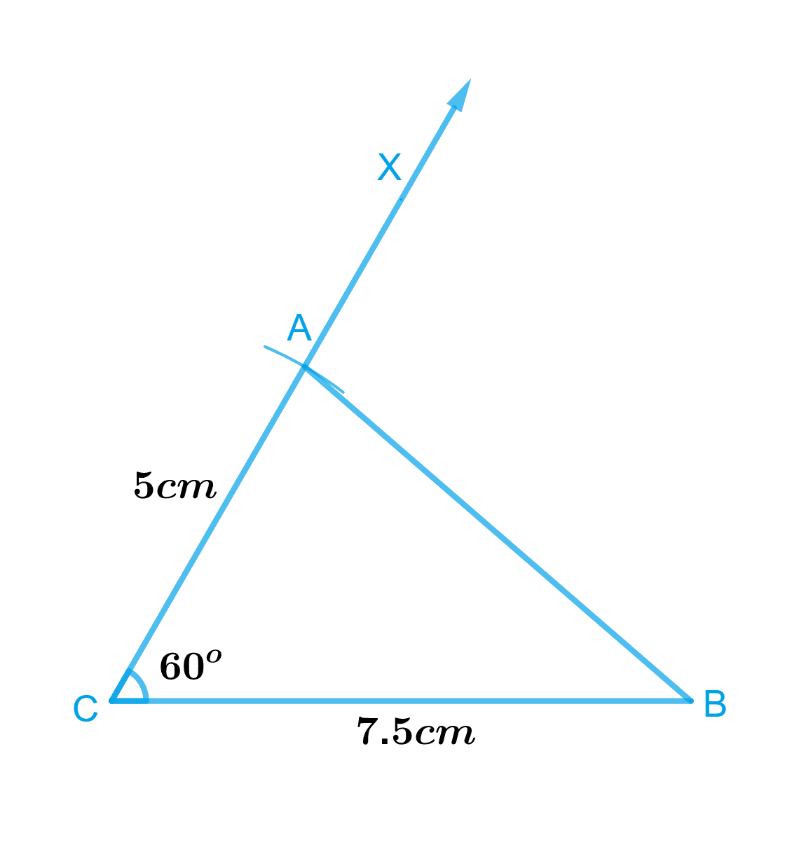# Ex.10.3 Q3 Practical Geometry Solution- NCERT Maths Class 7

Go back to  'Ex.10.3'

## Question

Construct $$\Delta ABC$$ with $$BC = 7.5\,\rm{ cm}$$, $$AC = 5\,\rm{ cm}$$ and $$m∠C = 60^\circ$$.

Video Solution
Practical Geometry
Ex 10.3 | Question 3

## Text Solution

What is known?

Lengths of sides of a triangle are $$BC = 7.5\,\rm{ cm}$$, $$AC = 5\,\rm{ cm}$$ and $$∠C = 60^\circ$$.

To construct:

A triangle $$\Delta ABC$$  with $$BC = 7.5\,\rm{ cm}$$, $$AC = 5\,\rm{ cm}$$ and $$∠C = 60^\circ$$.

Reasoning:

To construct a triangle $$ΔABC$$ with $$BC = 7.5\,{\rm{ cm}}, AC = 5\,\rm{ cm}$$ and $$∠C = 60^\circ$$, follow the steps given below.

Steps:Steps of construction :

1. Draw a line segment $$BC$$ of length $$7.5\,\rm{cm}.$$
2. At $$C$$, draw $$CX$$ making $$60^\circ$$ with $$BC$$.
3. With $$C$$ as centre, draw an arc of radius $$5\,\rm{cm}.$$ It cuts $$CX$$ at the point $$A$$.
4. Join $$AB.$$

Triangle $$ABC$$ is the required triangle.

Learn from the best math teachers and top your exams

• Live one on one classroom and doubt clearing
• Practice worksheets in and after class for conceptual clarity
• Personalized curriculum to keep up with school